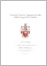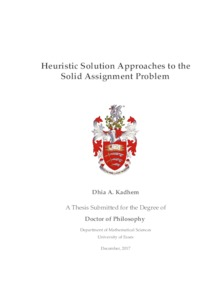# Heuristic Solution Approaches to the Solid Assignment Problem

Kadhem, Dhia (2017) Heuristic Solution Approaches to the Solid Assignment Problem. PhD thesis, University of Essex.Preview
Text
Dhia Thesis December.pdf

Download (3MB) | Preview

## Abstract

The 3-dimensional assignment problem, also known as the Solid Assignment Problem (SAP), is a challenging problem in combinatorial optimisation. While the ordinary or 2-dimensional assignment problem is in the P-class, SAP which is an extension of it, is NP-hard. SAP is the problem of allocating n jobs to n machines in n factories such that exactly one job is allocated to one machine in one factory. The objective is to minimise the total cost of getting these n jobs done. The problem is commonly solved using exact methods of integer programming such as Branch-and-Bound B&B. As it is intractable, only approximate solutions are found in reasonable time for large instances. Here, we suggest a number of approximate solution approaches, one of them the Diagonals Method (DM), relies on the Kuhn-Tucker Munkres algorithm, also known as the Hungarian Assignment Method. The approach was discussed, hybridised, presented and compared with other heuristic approaches such as the Average Method, the Addition Method, the Multiplication Method and the Genetic Algorithm. Moreover, a special case of SAP which involves Monge-type matrices is also considered. We have shown that in this case DM finds the exact solution efficiently. We sought to provide illustrations of the models and approaches presented whenever appropriate. Extensive experimental results are included and discussed. The thesis ends with a conclusions and some suggestions for further work on the same and related topics.

Item Type: Thesis (PhD) Q Science > QA Mathematics Faculty of Science and Health > Mathematical Sciences, Department of Dhia Kadhem 18 Jan 2018 10:18 05 Jan 2021 02:00 http://repository.essex.ac.uk/id/eprint/20907

### Actions (login required)View Item